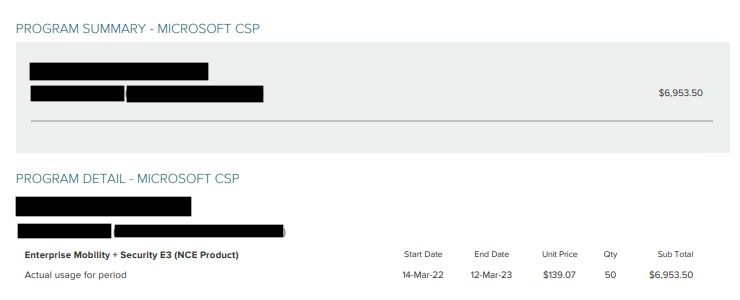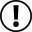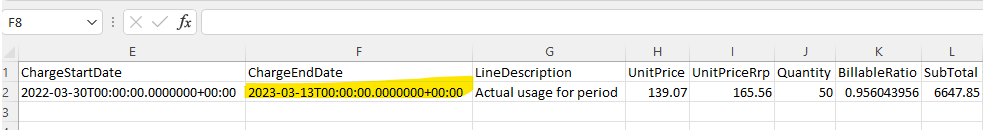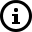# What are Pro-Rata Charges?

Pro rata is a Latin term – meaning “in proportion” – that is used to assign or allocate value in proportion to a billing period. For example a billing period of April 2022 (1st of April 2022 to 30th April 2022) and a pro-rata charge from 20th April 2022 to 30th April 2022.

## What is a billable ratio?

A billable ratio is a ratio of the number of days a subscription is active in the term to a specific period (usually a 1-month or 12-month period). The billable ratio formula is {Number Active Days}/{Total Days in Month}. Please note that the number of active days calculation should include the end date.

## How to calculate pro-rata charges?

We have created a calculator, that can be found here to assist you with calculating pro-rata charges under both monthly and annual termed subscriptions.  Please note that this calculator should be used in conjunction with the PRISM Price List.

## How is the billable ratio calculated?

### Monthly Term Subscriptions

Using the example that Customer ABC has a Microsoft 365 Business Basic subscription that was provisioned on the 3rd of April 2022 with a quantity of 10; and then on the 16th of April 2022 another 5 users were added to take the total to 15. The below example will use the example pricing of \$12.00 unit price.

Line 1: 3rd of April 2022 to 15th of April 2022 for 10
Number Active Days = 13 days
Total Days in Month = 30 days
Billable Ratio Formula = 13/30
Billable Ratio Result = 0.433333333
Line Item Formula = Unit Price * Quantity * Billable Ratio
Line Item Formula = ROUND(12.00 * 10 * 0.433333333,2)
Line Item Result = \$52.00

Line 2: 16th of April 2022 to 30th of April 2022 for 15
Number Active Days = 15 days
Total Days in Month = 30 days
Billable Ratio Formula = 15/30
Billable Ratio Result = 0.5
Line Item Formula = Unit Price * Quantity * Billable Ratio
Line Item Formula = ROUND(12.00 * 15 * 0.5,2)
Line Item Result = \$90.00

### Annual Term Subscriptions

Using the example that Customer ABC has a Microsoft 365 Business Standard subscription that was provisioned on the 14th of March 2022 with a quantity of 60; and then on the 24th of May 2022 another 45 users were added to take the total to 105. The below example will use the example pricing of \$115.00 unit price. As this is an annual term subscription the end date will be the 12th of March 2023 as the subscriptions end dates are inclusive. The exact number of days can be confirmed by inputting the dates displayed into free online tools such as dateandtime.com. This is shown for this example as 364 days. Ensure to tick 'Include end date in calculation'.

Invoice 1: 14th of March 2022 to 12th March 2023 for 60
Number Active Days = 364 days
Total Days in Term= 364 days
Billable Ratio Formula = 364/364
Billable Ratio Result = 1
Line Item Formula = Unit Price * Quantity * Billable Ratio
Line Item Formula = ROUND(115.00 * 60 * 1,2)
Line Item Result = \$6900.00

Invoice 2: 24th of May 2022 to 12th of March 2023 for 45
Number Active Days = 293 days
Total Days in Month = 364 days
Billable Ratio Formula = 293/364
Billable Ratio Result = 0.804945055
Line Item Formula = Unit Price * Quantity * Billable Ratio
Line Item Formula = ROUND(115.00 * 45 * 0.804945055,2)
Line Item Result = \$4165.59

## Understanding PRISM Data/Fields

Annual Invoice PDF - On the full term annual invoice, the subscription end date appears as the 12th of March 2022 (from the above example) as the dates are inclusive.Annual Invoice CSV - The 'ChargeEndDate' field inputs the expected date based on 365 days, unlike the PDF file where it implements the inclusivity.Note that the dates in the CSV do not correlate to the dates shown on the Invoice PDF. The end date displayed will be one day more than what is displayed on the PDF. In this example, the end date of (2023-03-13) is shown. This is because unlike all other invoice types, annual invoices store the subscription end date value. This obviously introduces a level of confusion and we apologise for this.

Unit price and billable ratio will appear as they should on the CSVDisclaimer - all pricing shown in this article is test data only and should not be used for quoting or purchasing decisions/information.